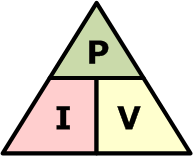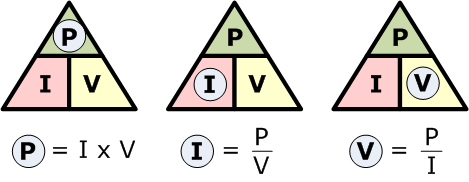<< index

by disinfoniacs #69 & #1

## power

>>>>

### tldr

• Power is the rate at which energy is transferred or transformed in an electrical circuit
• The power equation in a DC circuit is P=VI, where P is power, V is voltage, and I is current
• To calculate power, measure the voltage and current of the load, and multiply them together
• The acronym VIP or the phrase "Pizza Is Very Delicious" can be used to remember the formula for power in a DC circuit

## in depthIn an electrical circuit, power is the rate at which energy is transferred or transformed. It is measured in watts (W), and represents the amount of work that can be done by an electric current over a given period of time. In a DC circuit, the power delivered to a load can be calculated using the relationship P=VI, where P is power in watts, V is voltage in volts, and I is current in amps. This relationship is known as the power equation.

To understand this relationship, think of a circuit as a pipeline. Voltage is like the pressure in the pipeline, while current is like the flow of water. The power delivered by the pipeline is the product of the pressure and the flow rate. Similarly, in an electrical circuit, the power delivered to a load is the product of the voltage and the current.

To calculate power, first measure the voltage across the load and the current flowing through it. Then, multiply these two values together to obtain the power delivered to the load. For example, if a load has a voltage of 10 volts and a current of 2 amperes, the power delivered to the load is 20 watts.

To remember the formula for power in a DC circuit (P = IV), think of the acronym "VIP." In this case, "V" stands for voltage, "I" stands for current, and "P" stands for power. Just like a VIP at a party gets special treatment, voltage and current are the VIPs of electrical circuits, and power is the result of their interaction.

Another way to remember the formula is to use the phrase "Pizza Is Very Delicious." In this case, the first letter of each word represents a variable in the formula: "P" for power, "I" for current, and "V" for voltage. This phrase can help you remember the formula and the order of the variables.

---

<< index